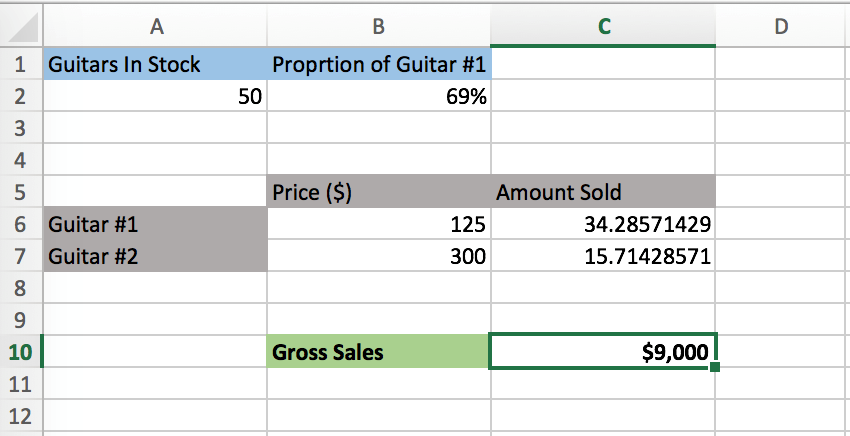# What-if Analysis in Excel – Scenario Manager & Goal Seek

The what-if analysis tools on Excel allow you to apply different values to formulas and compare results for a variety of scenarios.

## Why is it important to understand what-if analysis?

There are many applications of what-if analysis, such as financial modeling and sensitivity analyses. For example, if you are preparing a financial model, and you want to figure out how various revenue growth and EBIT margin assumptions affect a company’s earnings per share, a what-if analysis is necessary.

## How do I do a what-if analysis on Excel?

Let’s do a very basic example using the what-if analysis tools. Suppose you start your own music store, and you sell two types of guitars: guitar #1 which sells for \$125 and guitar #2 which sells for \$300. You realize that you have to cap the number of guitars in stock to 50. You can do a what-if analysis to figure out how the number of each guitar you keep in stock affects your store’s gross sales.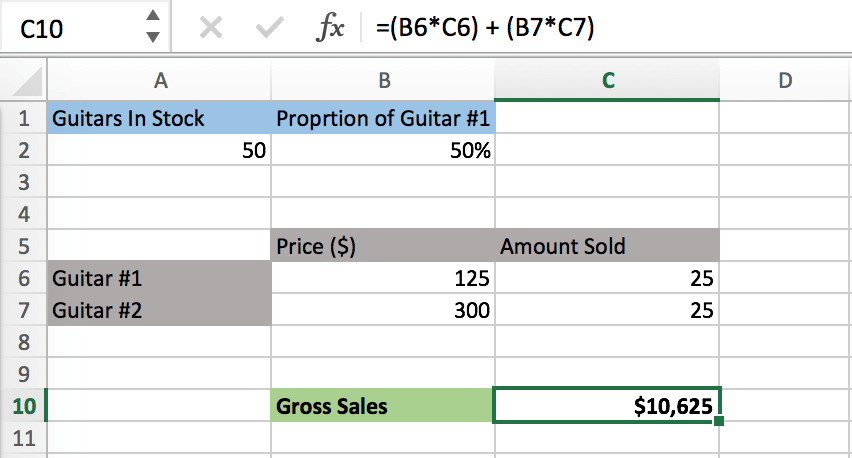When the proportion of Guitar #1 in stock is 50%, your gross sales are \$10,625, assuming that all guitars in stock are sold. To determine how Gross Sales are affected by Proportion of Guitar #1 using Excel’s what-if analysis tools, the formula for Gross Sales (C10) must be connected, either directly or indirectly, to Proportion of Guitar #1 (B2). In the example, Gross Sales is connected to Proportion of Guitar #1 because the formula for C10 is (B6*C6) + (B7*C7), the formula for C6 is B2*A2, and the formula for C7 is A2-C6; therefore, the Gross Sales formula indirectly includes B2.

What would the Gross Sales be if the Proportion of Guitar #1 was 25%? Or 75%? You can find out on Excel by selecting “Scenario Manager” under “What-If Analysis.”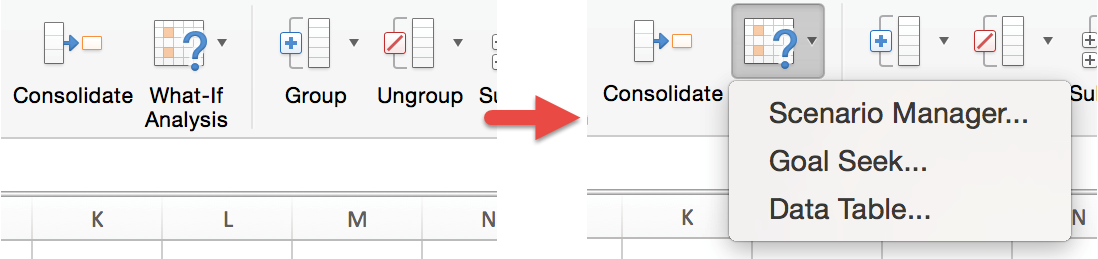After selecting scenario manager, you should end up on this screen: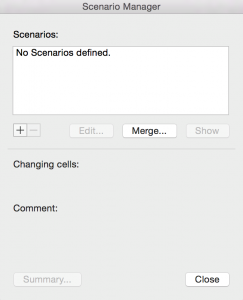Select “+” and this will show up: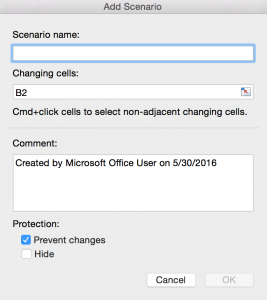It doesn’t matter what you write for “Scenario name” or “Comment,” but it is important that under “Changing cells” you put the cell(s) that you are testing. In this example, we are testing the Proportion of Guitar #1, which is B2. After you’re done, select “OK.”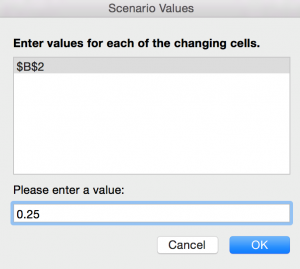Since we want to test how Gross Sales changes when the Proportion for Guitar #1 is 25%, enter 0.25 under “Please enter a value.”

After selecting “OK” you will see the original “Scenario Manager” screen with your new scenario included on the list. You can repeat the process for 75% and any other value of Proportion of Guitar #1 you want to test.

After you are done inserting your scenarios, select “Summary” on the bottom left of the “Scenario Manager” screen.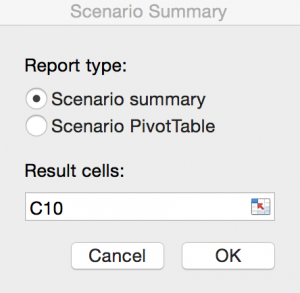You want the “Result cells” to be the dependent variable(s), which in this case is the Gross Sales (C10). Select “OK.”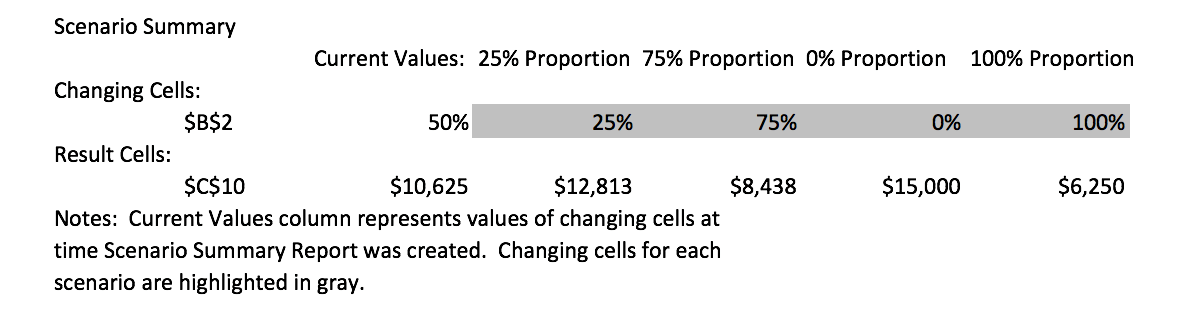Under the “Scenario Summary,” you can see all your scenarios for different Proportions of Guitar #1 and their corresponding Gross Sales value.

The Goal Seek feature, which is one of Excel’s what-if analysis tools, allows you to input a value for a dependent variable, and then calculates the corresponding value for the independent variable. Referring to the music store example, if you want to determine what proportion of guitar #1 you have to keep in stock to make \$9,000 in gross sales. Select “Goal Seek” under “What-If Analysis.”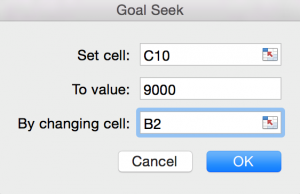Under “Set cell” is the cell with the dependent variable (Gross Sales), under “To value” is the value that you want to assign to the dependent variable, and under “By changing cell” is the cell with the independent variable (Proportion of Guitar #1). Click “OK,” and you will see the corresponding value for the independent variable on your spreadsheet.